# AWS TypeScript CDK and Step Functions

## How to deploy a TypeScript step function composed of 3 lambdas.This quick tutorial will show the steps for deploying a TypeScript step function composed of 3 lambdas. 1 lambda generates a random number, there is a 1-second wait then in the step function. Then there is a check if the generated number is greater or less than a pre-assigned number which is passed in when we invoke the step function. If the number is greater, 1 lambda is invoked, if it is less than or equal to that number, a different lambda is invoked.

There is no huge business case for a step function like this but it's a starter for implementing step functions using the TypeScript CDK.

This is an introductory tutorial and more work would be needed to make this production-ready but this tutorial will hopefully get you on your way.

Step 1: Install AWS CLI

Follow the steps on the following AWS Page to install the AWS — https://docs.aws.amazon.com/cli/latest/userguide/install-cliv2.html

If the AWS CLI install is successful, running the following command should yield results

``````aws --version
``````

This should output results similar to the following (dependent on your OS and the version of the aws cli installed). If the AWS cli is not installed successfully, you will get a warning message

``````aws-cli/2.1.16
``````

Step 2: Configure the AWS CLI

Follow the steps on the following AWS Page to configure the AWS CLI — https://docs.aws.amazon.com/cli/latest/userguide/cli-configure-quickstart.html#cli-configure-quickstart-config

This is important as it tells the AWS cli which aws account and region to use.

Step 3: Install Node.js

NodeJs is required as this is a typescript project and we will be using the typescript CDK.

Install NodeJs by following the instructions on the NodeJS website — https://nodejs.org/en/

Running the following command:

``````node --version
``````

should yield results similar to the following (dependent on your OS and the version of node installed)

``````v14.17.3
``````

Step 4: Install the AWS CDK

The AWS typescript cdk is required to work with the CDK.

Run the following command to install the CDK:

``````npm install -g aws-cdk
``````

To validate the CDK is installed correctly, run the following command:

``````cdk --version
``````

This should yield results similar to the following (dependent on your OS and CDK version installed):

``````1.115.0 (build f0ca40f)
``````

Step 5: Setup CDK Project

Run the following command to create a new folder and cd into it:

``````mkdir cdk-statemachine && cd cdk-statemachine
``````

Run the following command to initiate the CDK project:

``````cdk init app --language typescript
``````

In a separate terminal window, run the watch command which will keep track of changes as we make them to the project:

``````npm run watch
``````

Step 6: Install Lambda Constructs

The AWS CDK Lamda construct is required as we want to deploy a Lambda to our AWS accounts.

To install the aws-lambda construct, run the following command:

``````npm install @aws-cdk/aws-lambda
``````

We also need to install a few more aws constructs that will be used for this tutorial — aws-stepfunctions and aws-stepfunctions-tasks

Run the following command to install these contructs:

``````npm install @aws-cdk/aws-stepfunctions
``````

Step 7: Create Required Lambdas

As mentioned there will be three lambdas required for this project:

1. One lambda generates a random number between 1 and a max pre defined number

2. Another lambda is called if the generated number is greater than a pre defined number to check

3. Another lambda is called if the generated number is less than or equal to a pre defined number to check

Create a folder in the root of your project (same level as bin and lib) called lambda.

Add a file to the lambda folder called generateRandomNumber.js (lambda/generateRandomNumber.js)

Inside generateRandomNumber.js — add the following content:

``````exports.handler = (event, context, callback) => {
const generateRandom = (maxNumber) => Math.floor(Math.random() * maxNumber) + 1;
callback(null, {
"generatedRandomNumber": generateRandom(event.maxNumber),
"maxNumber": parseInt(event.maxNumber),
"numberToCheck": parseInt(event.numberToCheck)
});
}
``````

Add a file to the lambda folder called greater.js (lambda/greater.js).

Inside greater.js — add the following content:

``````exports.handler = (event, context, callback) => {
callback(null, {"msg": "greater"});
};
``````

Add a file to the lambda folder called greater.js (lambda/lessOrEqual.js).

Inside lessOrEqual.js — add the following content:

``````exports.handler = (event, context, callback) => {
callback(null, {"msg": "lessOrEqual"});
};
``````

This code is purely to demo the functionality offered by the CDK and deploying a stap function. Further work is required to make it production ready.

Step 8: Add lambdas to stack

We have now added our lambda code, but as of yet, it will not be picked up by the CDK.

In your favourite text editor, open the file lib/cdk-statemachine-stack.ts

At the top of the file, add the aws-lambda construct import below the aws-cdk/core import by adding this line:

``````import * as lambda from '@aws-cdk/aws-lambda';
import * as sfn from '@aws-cdk/aws-stepfunctions';
``````

Add the following line just above the constructor definition:

``````public Machine: sfn.StateMachine;
``````

Replace the line

``````// The code that defines your stack goes here
``````

with the following code:

``````// Lambda to generate a random number
const generateRandomNumber = new lambda.Function(this, 'GenerateRandomNumber', {
runtime: lambda.Runtime.NODEJS_14_X,
code: lambda.Code.fromAsset('lambda'),
handler: 'generateRandomNumber.handler',
timeout: cdk.Duration.seconds(3)
});

//Lambda invocation for generating a random number
const generateRandomNumberInvocation = new tasks.LambdaInvoke(this, 'Generate random number invocation', {
lambdaFunction: generateRandomNumber,
});

// Lambda function called if the generated number is greater than the expected number
const functionGreaterThan = new lambda.Function(this, "NumberGreaterThan", {
runtime: lambda.Runtime.NODEJS_14_X,
code: lambda.Code.fromAsset('lambda'),
handler: 'greater.handler',
timeout: cdk.Duration.seconds(3)
});

// Lambda invocation if the generated number is greater than the expected number
const greaterThanInvocation = new tasks.LambdaInvoke(this, 'Get Number is greater than invocation', {
lambdaFunction: functionGreaterThan,
inputPath: '\$',
outputPath: '\$',
});

// Lambda function called if the generated number is less than or equal to the expected number
const functionLessThanOrEqual = new lambda.Function(this, "NumberLessThan", {
runtime: lambda.Runtime.NODEJS_14_X,
code: lambda.Code.fromAsset('lambda'),
handler: 'lessOrEqual.handler',
timeout: cdk.Duration.seconds(3)
});

// Lambda invocation if the generated number is less than or equal to the expected number
const lessThanOrEqualInvocation = new tasks.LambdaInvoke(this, 'Get Number is less than or equal invocation', {
lambdaFunction: functionLessThanOrEqual,
inputPath: '\$',
outputPath: '\$',
});

// Lambda invocation if the generated number is less than or equal to the expected number
const lessThanOrEqualInvocation = new tasks.LambdaInvoke(this, 'Get Number is less than or equal invocation', {
lambdaFunction: functionLessThanOrEqual,
inputPath: '\$',
outputPath: '\$',
});
``````

This code tells the CDK to deploy the lambdas that we previously created to our AWS Account. The lambda invoke statements are required for the state machine and are used to invoke the lambda as a task.

Step 9: Install Step Function

This snippet of code is used to install the actual step function which will use the lambdas that were created previously.

``````//Condition to wait 1 second
const wait1Second = new sfn.Wait(this, "Wait 1 Second", {
time: sfn.WaitTime.duration(cdk.Duration.seconds(1)),
});

//Choice condition for workflow
const numberChoice = new sfn.Choice(this, 'Job Complete?')
.when(sfn.Condition.numberGreaterThanJsonPath('\$.generatedRandomNumber', '\$.numberToCheck'), greaterThanInvocation)
.when(sfn.Condition.numberLessThanEqualsJsonPath('\$.generatedRandomNumber', '\$.numberToCheck'), lessThanOrEqualInvocation).otherwise(lessThanOrEqualInvocation);

//Create the workflow definition
const definition = generateRandomNumberInvocation.next(wait1Second).next(numberChoice);

//Create the statemachine
this.Machine = new sfn.StateMachine(this, "StateMachine", {
definition,
stateMachineName: 'randomNumberStateMachine',
timeout: cdk.Duration.minutes(5),
});
``````

The first part creates a wait step — just purely to demonstrate how this step is added to the step function.

As mentioned previously, there is a choice step which invokes the correct lambda depending on the random number generated.

The definition ties all this together where the random number lambda is called followed by the wait and finally the lambda which says whether the random number is greater than or less than the number we passed to the step function is called.

Finally the last piece of code is what creates the Step Function / State machine.

The final version of the cdk-statemachine-stack.ts should look like this:

``````import * as cdk from '@aws-cdk/core';
import * as lambda from '@aws-cdk/aws-lambda';
import * as sfn from '@aws-cdk/aws-stepfunctions';

export class CdkStatemachineStack extends cdk.Stack {

public Machine: sfn.StateMachine;
constructor(scope: cdk.Construct, id: string, props?: cdk.StackProps) {
super(scope, id, props);

// Lambda to generate a random number
const generateRandomNumber = new lambda.Function(this, 'GenerateRandomNumber', {
runtime: lambda.Runtime.NODEJS_14_X,
code: lambda.Code.fromAsset('lambda'),
handler: 'generateRandomNumber.handler',
timeout: cdk.Duration.seconds(3)
});

//Lambda invocation for generating a random number
const generateRandomNumberInvocation = new tasks.LambdaInvoke(this, 'Generate random number invocation', {
lambdaFunction: generateRandomNumber,
});

// Lambda function called if the generated number is greater than the expected number
const functionGreaterThan = new lambda.Function(this, "NumberGreaterThan", {
runtime: lambda.Runtime.NODEJS_14_X,
code: lambda.Code.fromAsset('lambda'),
handler: 'greater.handler',
timeout: cdk.Duration.seconds(3)
});

// Lambda invocation if the generated number is greater than the expected number
const greaterThanInvocation = new tasks.LambdaInvoke(this, 'Get Number is greater than invocation', {
lambdaFunction: functionGreaterThan,
inputPath: '\$',
outputPath: '\$',
});

// Lambda function called if the generated number is less than or equal to the expected number
const functionLessThanOrEqual = new lambda.Function(this, "NumberLessThan", {
runtime: lambda.Runtime.NODEJS_14_X,
code: lambda.Code.fromAsset('lambda'),
handler: 'lessOrEqual.handler',
timeout: cdk.Duration.seconds(3)
});

// Lambda invocation if the generated number is less than or equal to the expected number
const lessThanOrEqualInvocation = new tasks.LambdaInvoke(this, 'Get Number is less than or equal invocation', {
lambdaFunction: functionLessThanOrEqual,
inputPath: '\$',
outputPath: '\$',
});

//Condition to wait 1 second
const wait1Second = new sfn.Wait(this, "Wait 1 Second", {
time: sfn.WaitTime.duration(cdk.Duration.seconds(1)),
});

//Choice condition for workflow
const numberChoice = new sfn.Choice(this, 'Job Complete?')
.when(sfn.Condition.numberGreaterThanJsonPath('\$.generatedRandomNumber', '\$.numberToCheck'), greaterThanInvocation)
.when(sfn.Condition.numberLessThanEqualsJsonPath('\$.generatedRandomNumber', '\$.numberToCheck'), lessThanOrEqualInvocation).otherwise(lessThanOrEqualInvocation);

//Create the workflow definition
const definition = generateRandomNumberInvocation.next(wait1Second).next(numberChoice);

//Create the statemachine
this.Machine = new sfn.StateMachine(this, "StateMachine", {
definition,
stateMachineName: 'randomNumberStateMachine',
timeout: cdk.Duration.minutes(5),
});
}

}
``````

Step 10: Setup AWS Environment For CDK

The very first time you install a CDK stack to an AWS environment, you need to set it up with a bootstrap stack.

This stack can be installed by running the following command:

``````cdk bootstrap
``````

Step 11: Deploy Random Number Step Function to AWS Account

This step will take all the code we just worked with and deploy it to our AWS Account.

We simply need to just run the following command to tell the CDK to deploy our changes:

``````cdk deploy
``````

When prompted to accept the changes — enter y.

Step 12: Test functionality

In this section, we will use the Step Functions UI on the AWS console to test what we have deployed.

Navigate to the Step Functions Screen.

Select the state machine called randomNumberStateMachine.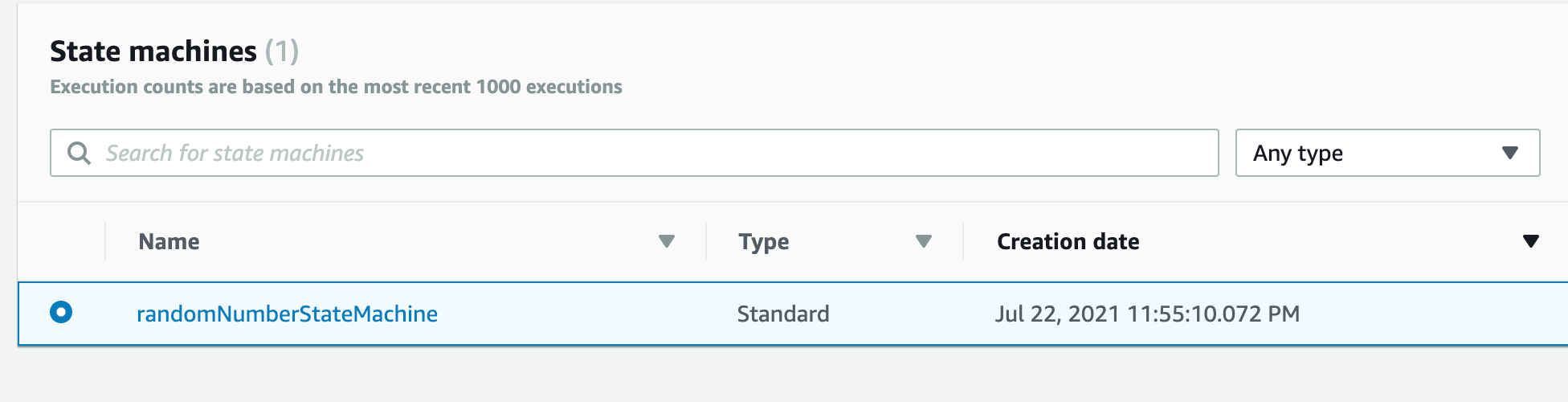Click button on top right called “Start Execution”.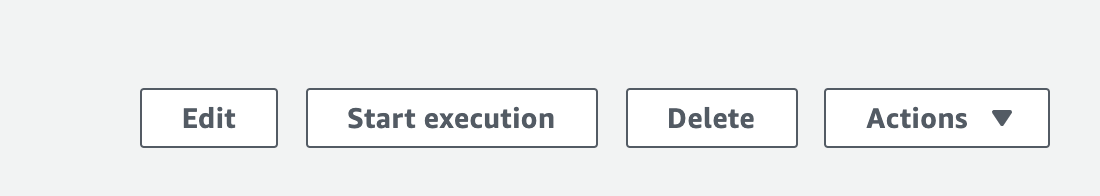For input — enter the following text:

``````{
"maxNumber": 10,
"numberToCheck": 7
}
``````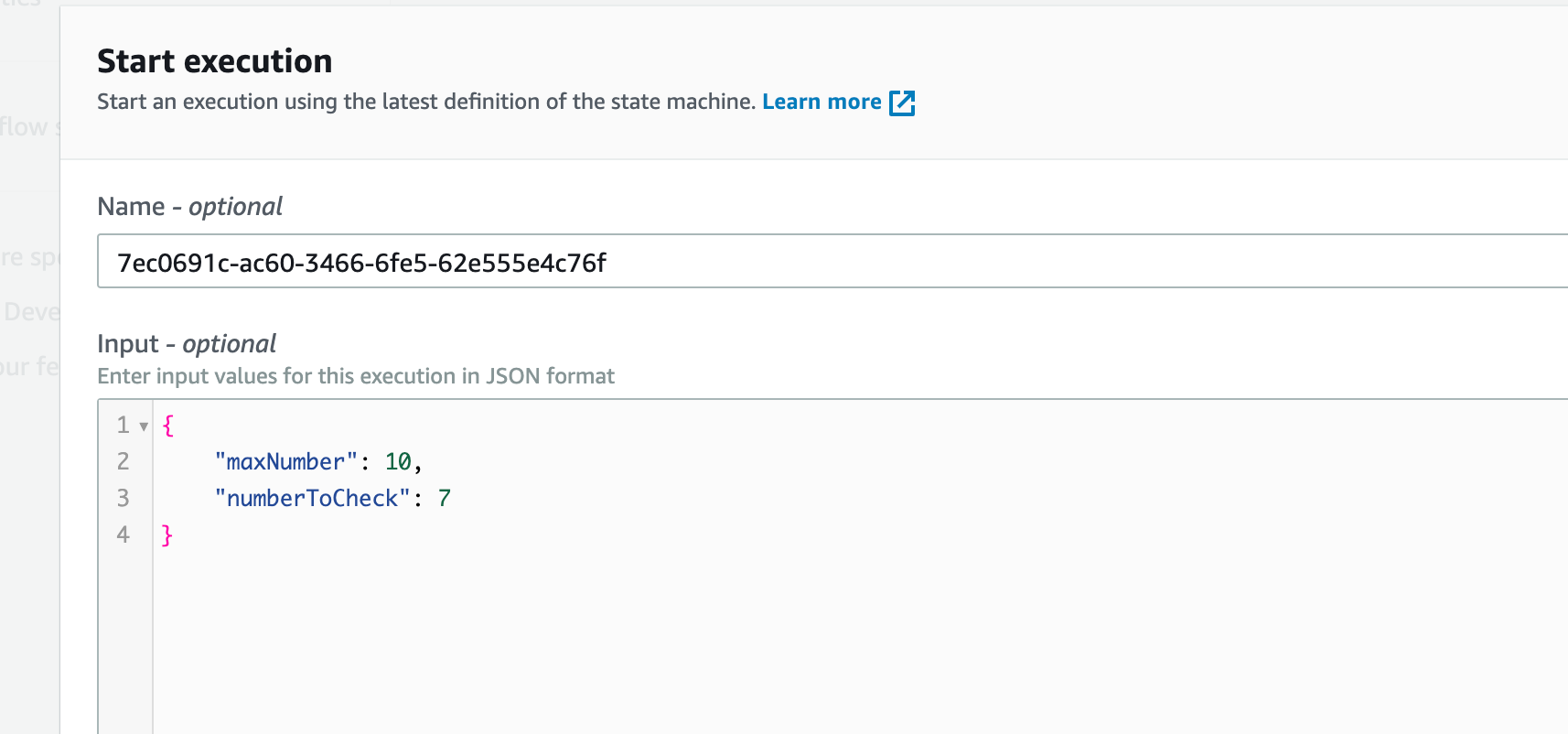Click Start Execution on the bottom right of the screen.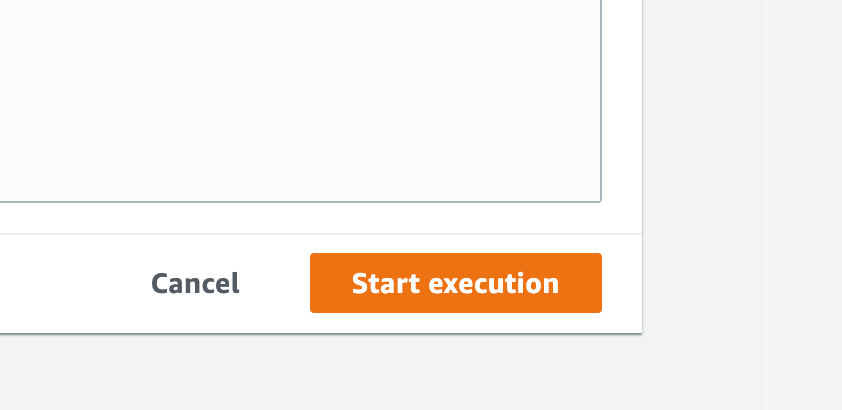If the generated number is less than or equal to the random number generated, the graph inspector will look like this: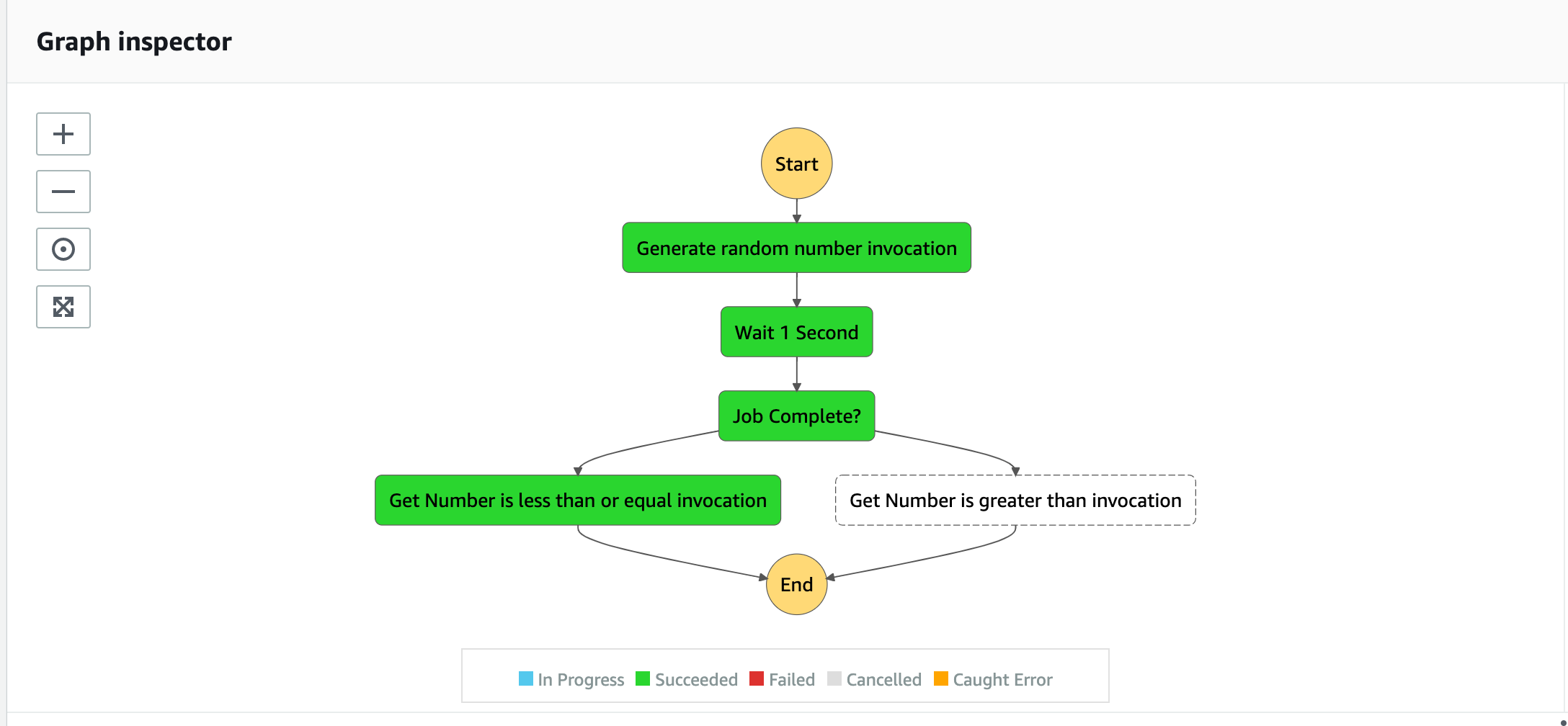If the generated number is greater than the random number generated, the graph inspector will look like this: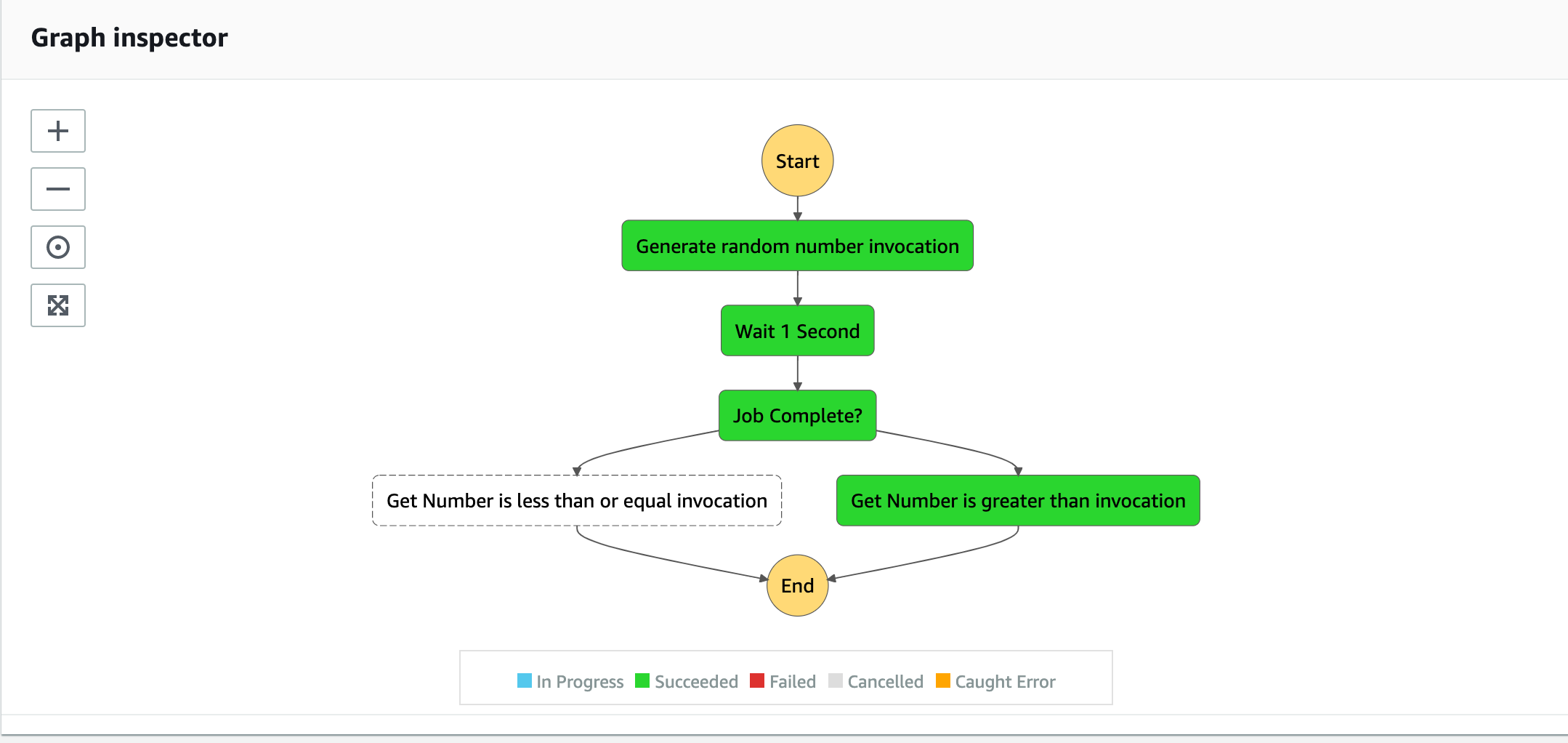Step 13: Delete AWS Resources

Now that this tutorial is complete, you may want to remove the resources in order to keep your AWS account tidy.

To delete all generated AWS resources from your account, run the following command:

``````cdk destroy
``````

When prompted to accept that you want to delete the resources — enter y.

Conclusion

This tutorial showed how to set up the AWS TypeScript CDK and deploy a random number checker to an AWS Step Function.

As mentioned at the start, this code is not production-ready and is just an intro tutorial.

If you wish to view the code for this tutorial — it is available here — https://github.com/damogallagher/typescript-stepfunctions-cdk/tree/develop.

Note: There will be a follow-up tutorial in the near future showing how to trigger this step function using the API Gateway. The link will be added to the bottom of this tutorial when that is available.